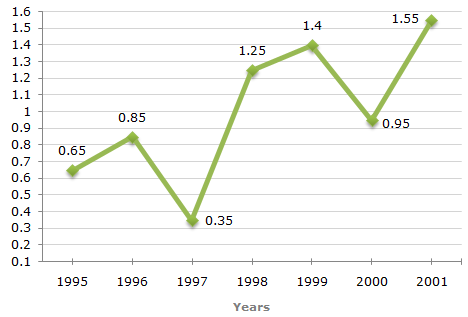# Data Interpretation - Line Charts - Discussion

### Discussion :: Line Charts - Line Chart 2 (Q.No.2)

The following line graph gives the ratio of the amounts of imports by a company to the amount of exports from that company over the period from 1995 to 2001.

Ratio of Value of Imports to Exports by a Company Over the Years.2.

The imports were minimum proportionate to the exports of the company in the year ?

 [A]. 1995 [B]. 1996 [C]. 1997 [D]. 2000

Explanation:

The imports are minimum proportionate to the exports implies that the ratio of the value of imports to exports has the minimum value.

Now, this ratio has a minimum value 0.35 in 1997, i.e., the imports are minimum proportionate to the exports in 1997.

 Anitha said: (Aug 14, 2015) Given that ratio of imports and exports were given, but the answer is the least percent (0.35). How?

 Kiran8949 said: (Sep 6, 2019) Import is directly proportional to ratio. So, the least ration will be the least import.

 Nidup Sanji said: (Oct 31, 2020) Minimum proportionate means less proportionate meaning more difference between imports and exports. More the exports compared to imports lesser values. Ex. 1/2=0. 5 and 1/3=0. 33. Clearly, it shows that exports (denominator) are inversely proportional that is less proportionate.